# MSBSHSE Solutions For Class 8 Maths Part 2 Chapter 16 Surface Area and Volume

MSBSHSE Solutions For Class 8 Maths Part 2 Chapter 16 Surface area and Volume can be accessed here, so that students can prepare well and excel in their exams. This chapter focuses on the concept of Surface area and Volume. Finding the surface area and volume of different solids are well explained. Subject experts at BYJU’S have prepared the Maharashtra Board Solutions for Class 8, in a simple language for students to understand the concepts at ease. The detailed solutions to the exercise problems in Maharashtra State Board Class 8 Textbook Part 2 are given here. The Maharashtra State Board Solutions for Chapter 16 are now available in pdf format and students can download it, from the link given below. This resource can be used for any quick reference.

## Download the PDF of Maharashtra Board Solutions For Class 8 Maths Part 2 Chapter 16 Surface area and Volume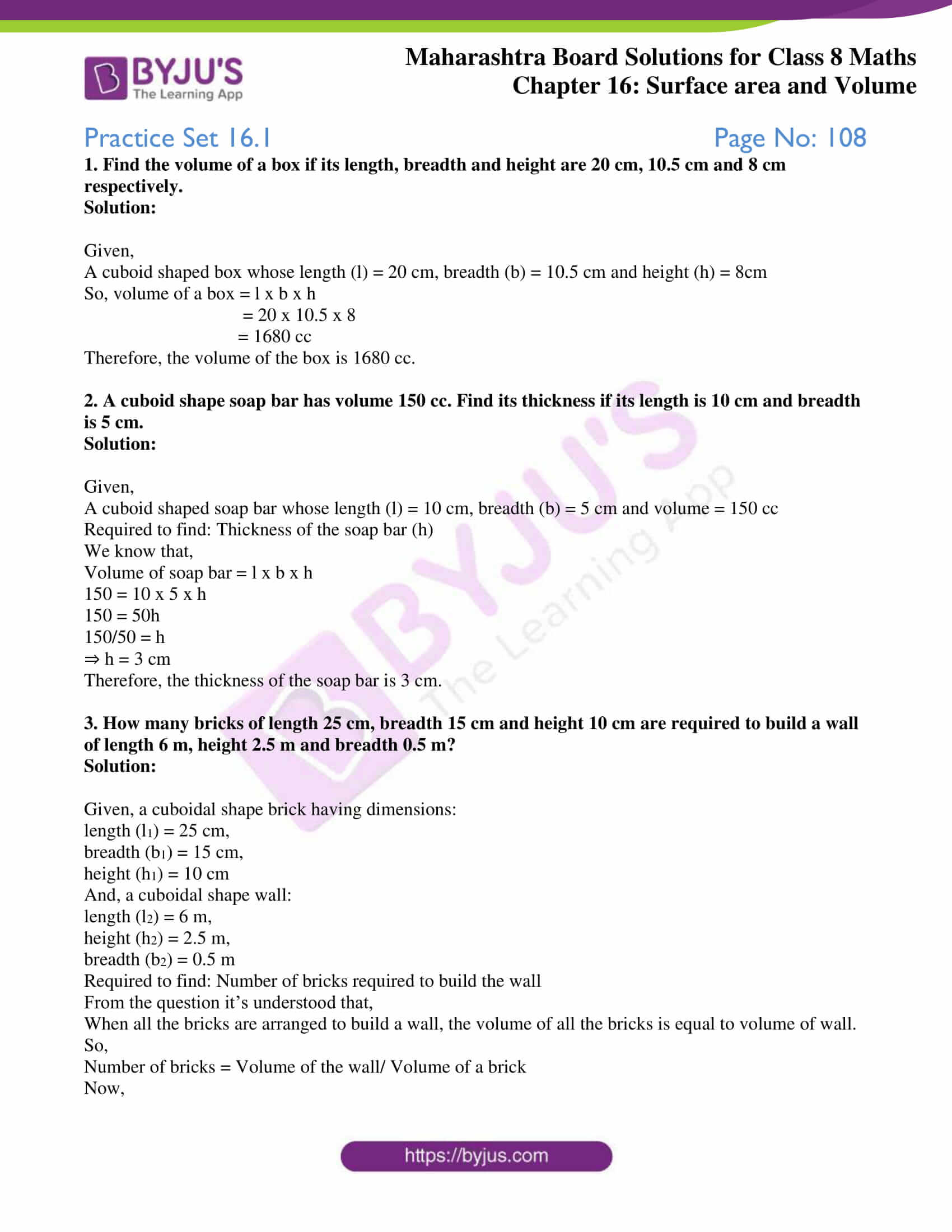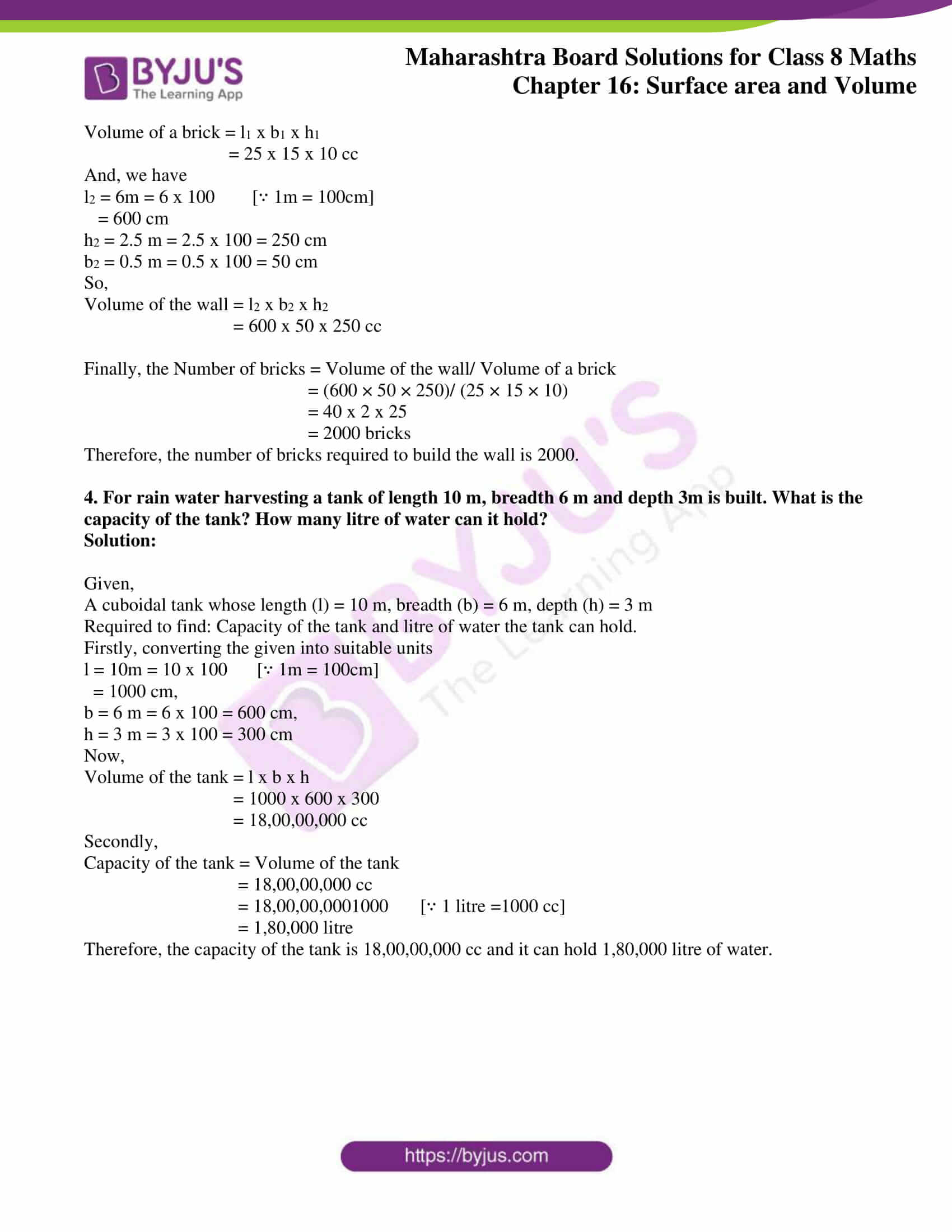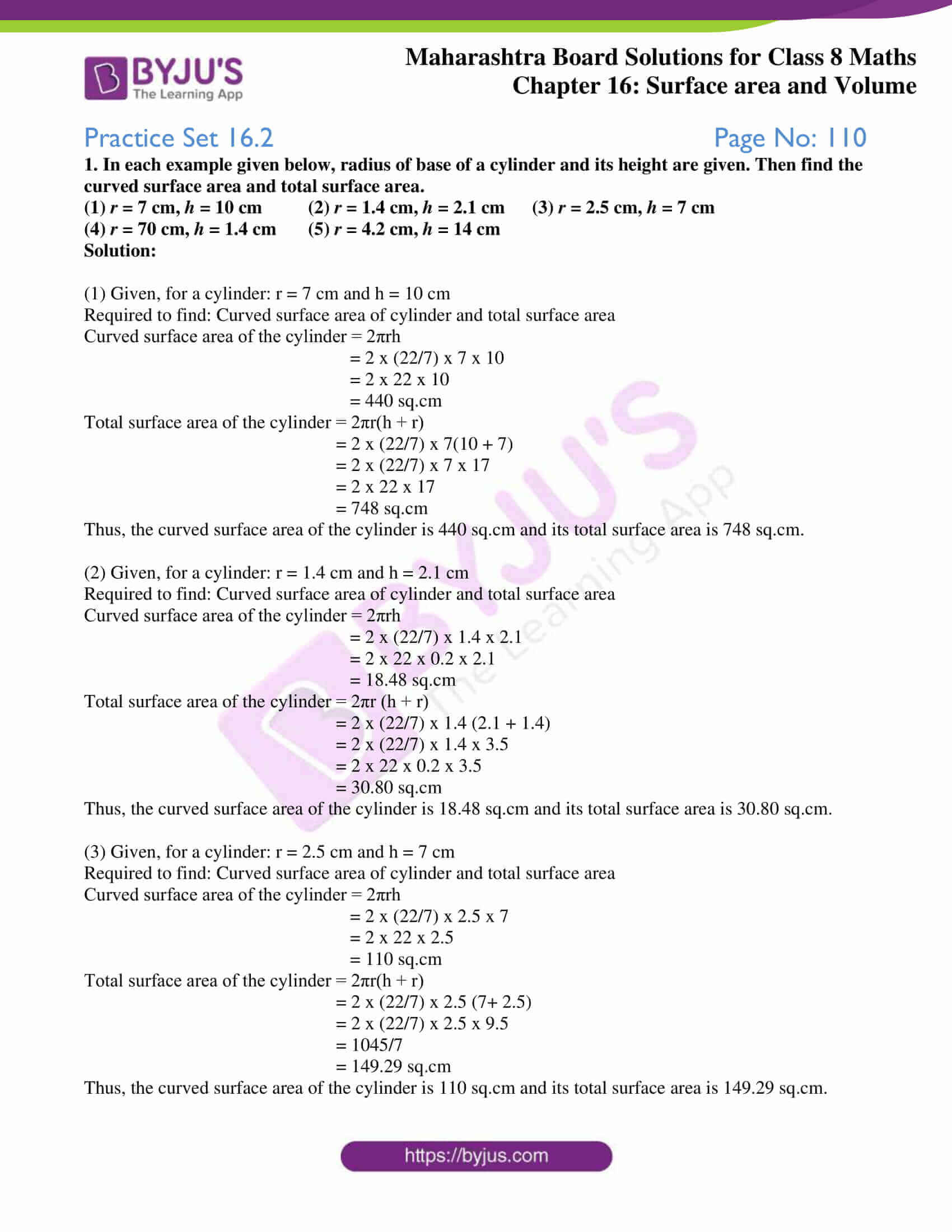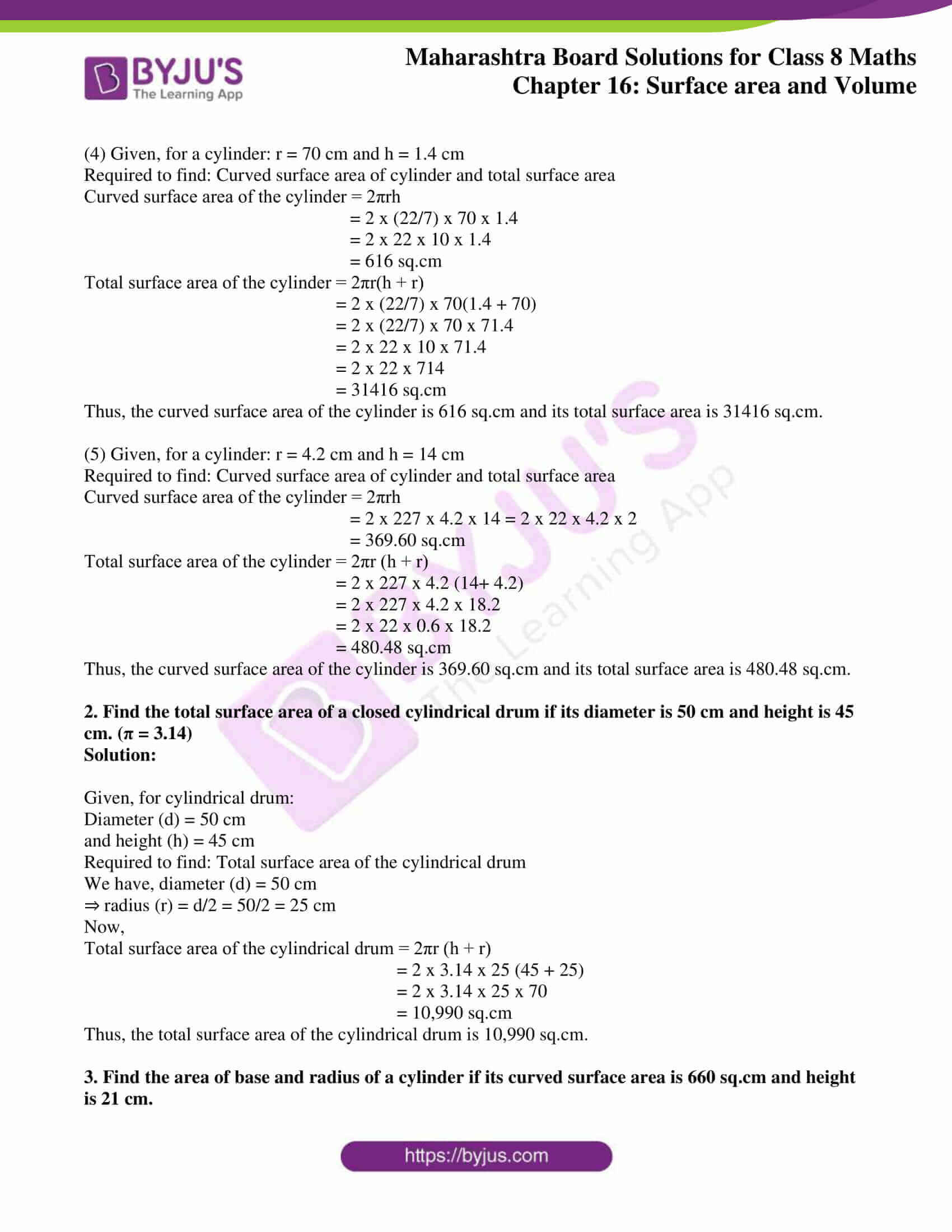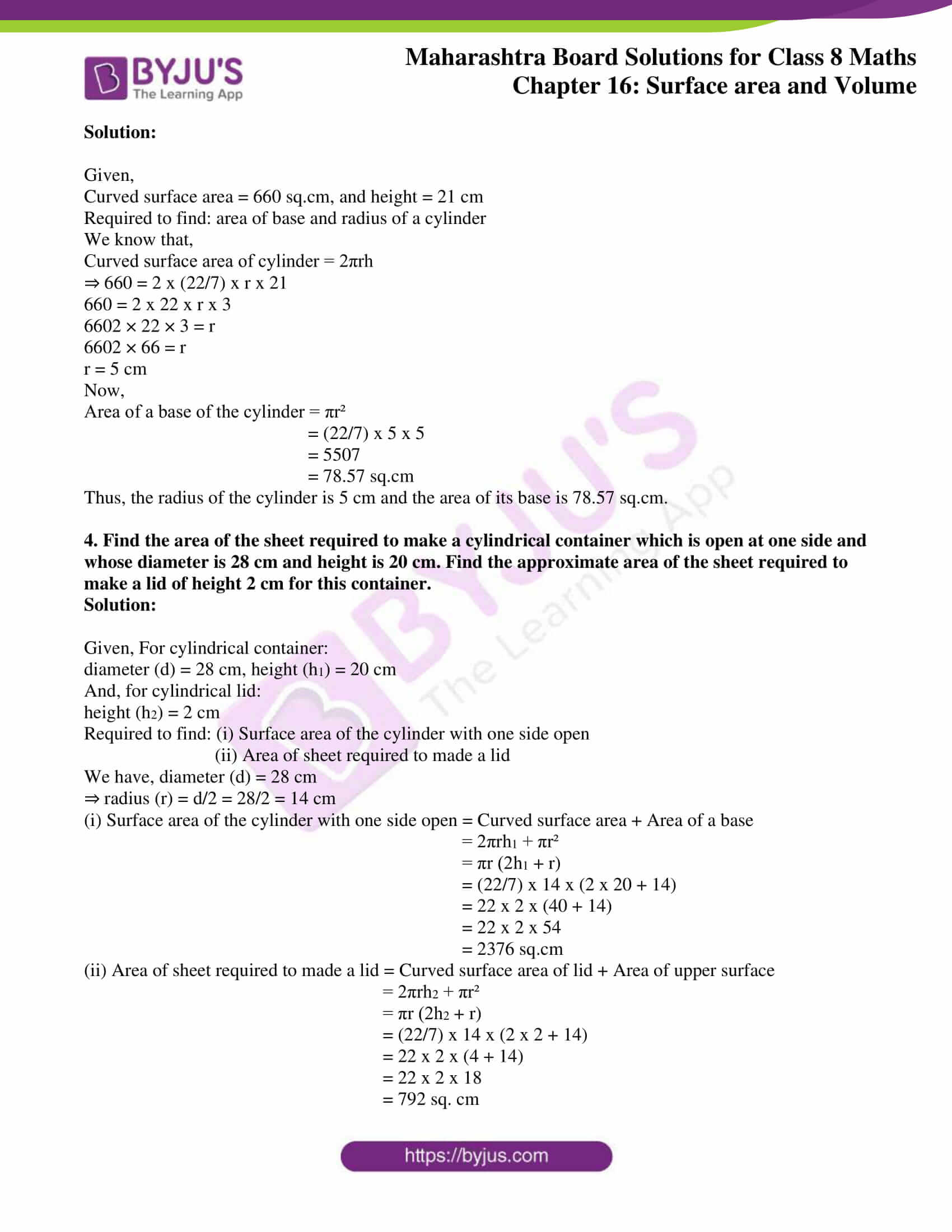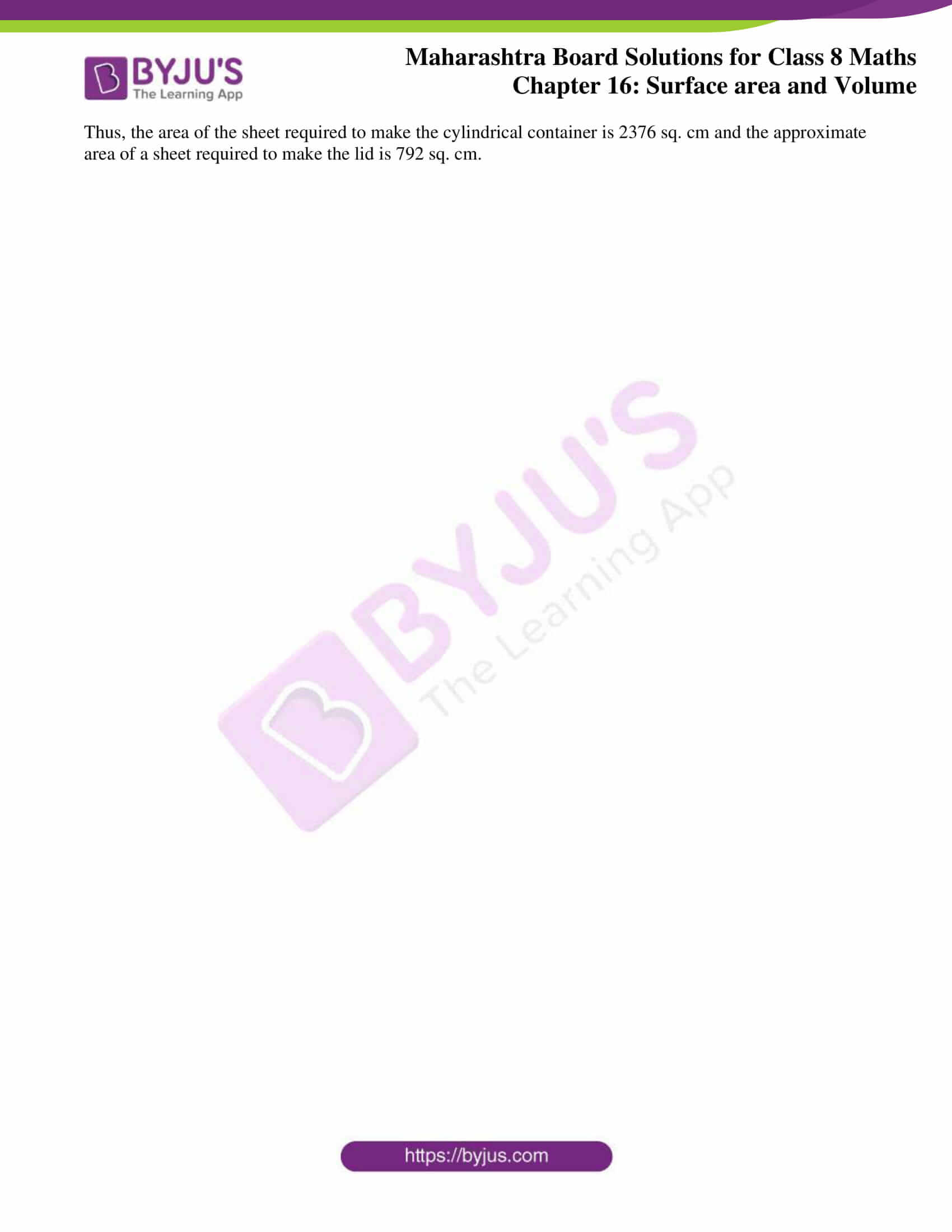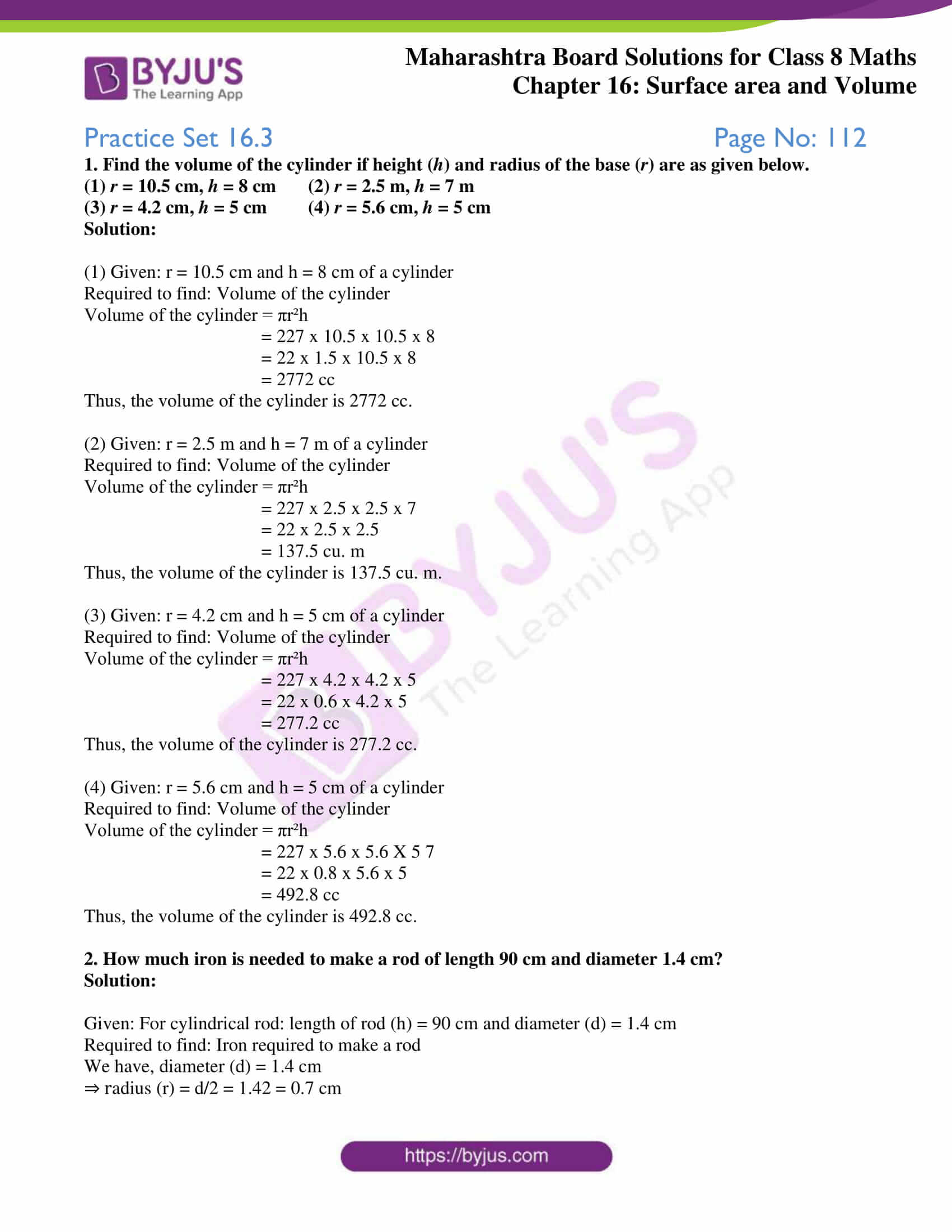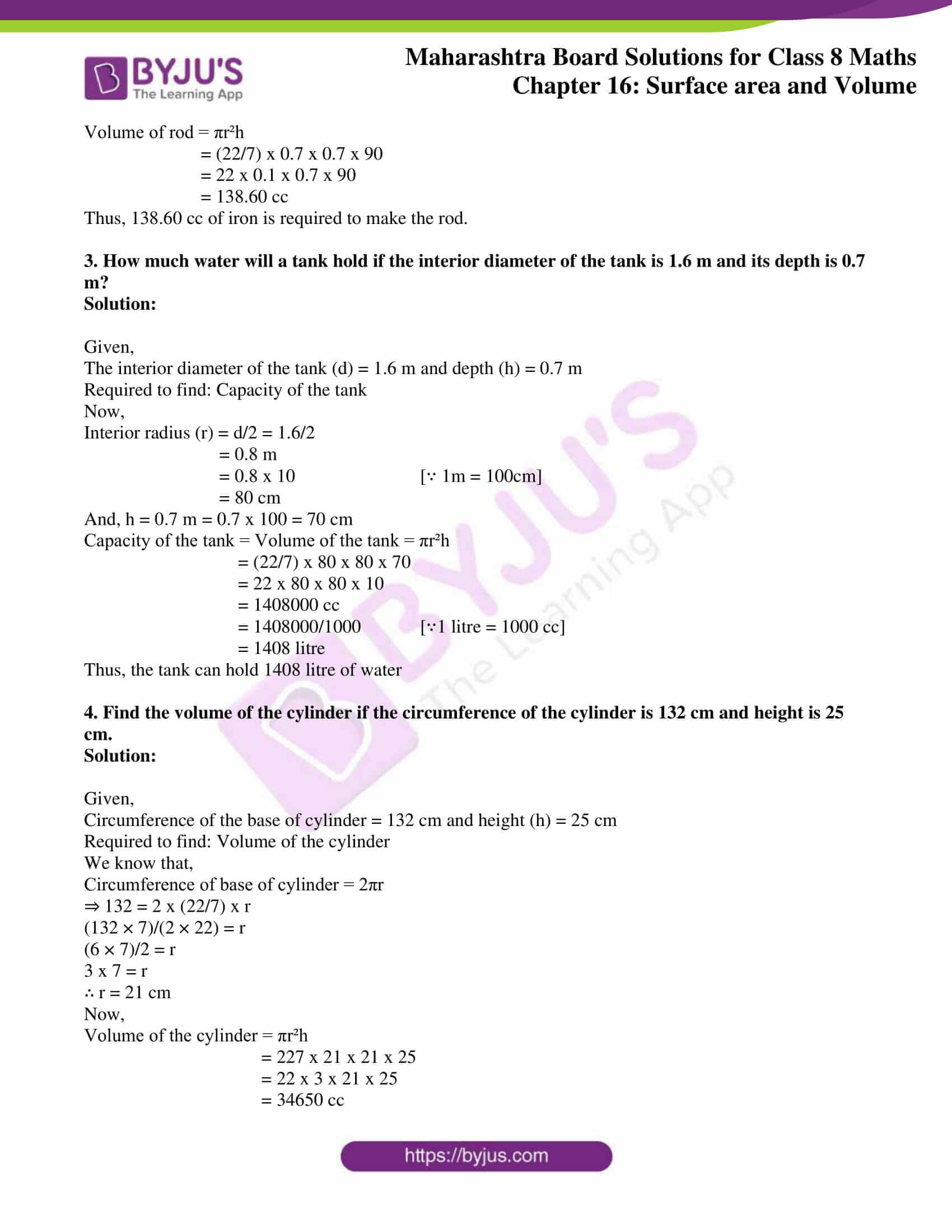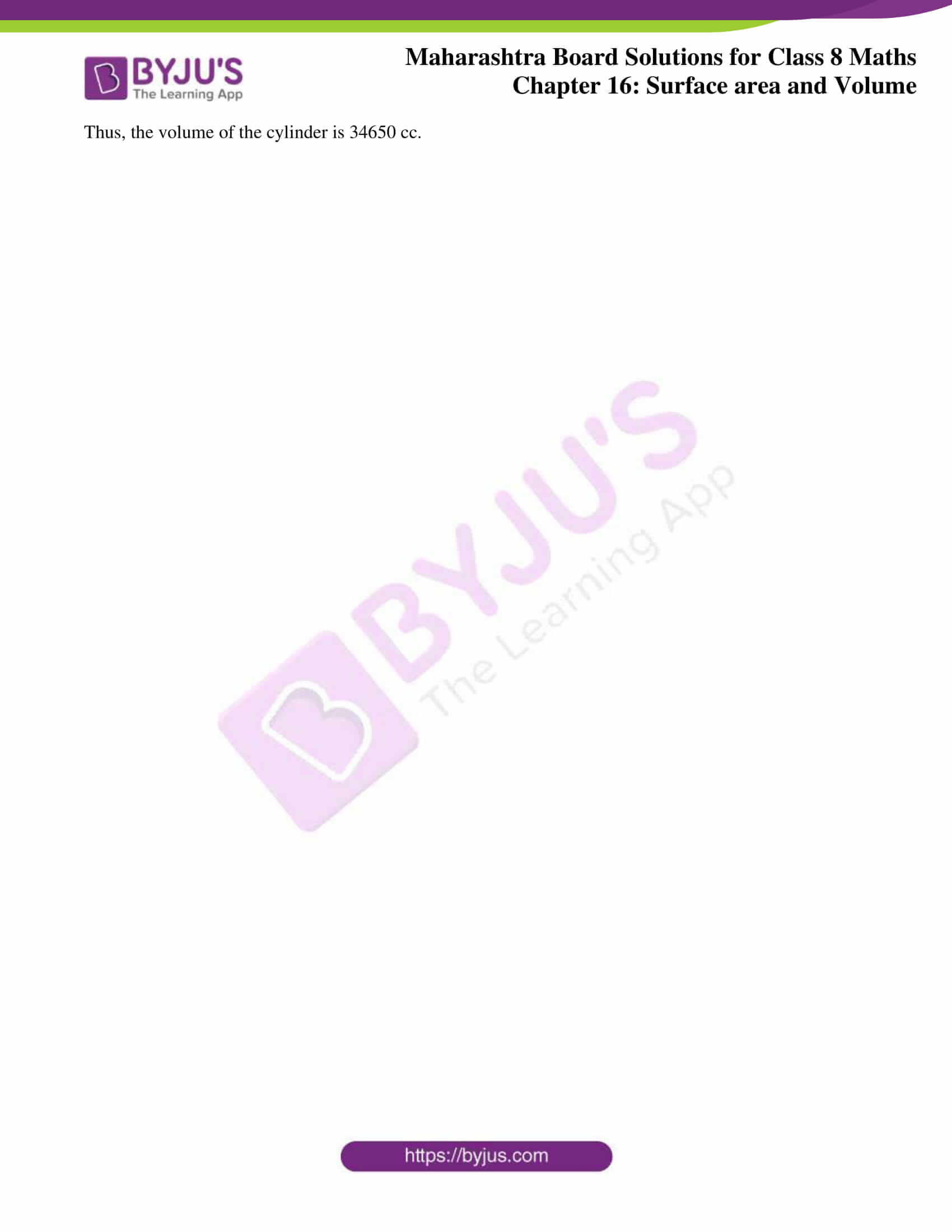### Access answers to Maharashtra Board Solutions For Class 8 Maths Part 2 Chapter 16 Surface area and Volume

Practice Set 16.1 Page No: 108

1. Find the volume of a box if its length, breadth and height are 20 cm, 10.5 cm and 8 cm respectively.

Solution:

Given,

A cuboid shaped box whose length (l) = 20 cm, breadth (b) = 10.5 cm and height (h) = 8cm

So, volume of a box = l x b x h

= 20 x 10.5 x 8

= 1680 cc

Therefore, the volume of the box is 1680 cc.

2. A cuboid shape soap bar has volume 150 cc. Find its thickness if its length is 10 cm and breadth is 5 cm.

Solution:

Given,

A cuboid shaped soap bar whose length (l) = 10 cm, breadth (b) = 5 cm and volume = 150 cc

Required to find: Thickness of the soap bar (h)

We know that,

Volume of soap bar = l x b x h

150 = 10 x 5 x h

150 = 50h

150/50 = h

⇒ h = 3 cm

Therefore, the thickness of the soap bar is 3 cm.

3. How many bricks of length 25 cm, breadth 15 cm and height 10 cm are required to build a wall of length 6 m, height 2.5 m and breadth 0.5 m?

Solution:

Given, a cuboidal shape brick having dimensions:

length (l1) = 25 cm,

height (h1) = 10 cm

And, a cuboidal shape wall:

length (l2) = 6 m,

height (h2) = 2.5 m,

Required to find: Number of bricks required to build the wall

From the question it’s understood that,

When all the bricks are arranged to build a wall, the volume of all the bricks is equal to volume of wall.

So,

Number of bricks = Volume of the wall/ Volume of a brick

Now,

Volume of a brick = l1 x b1 x h1

= 25 x 15 x 10 cc

And, we have

l2 = 6m = 6 x 100 [∵ 1m = 100cm]
= 600 cm

h2 = 2.5 m = 2.5 x 100 = 250 cm

b2 = 0.5 m = 0.5 x 100 = 50 cm

So,

Volume of the wall = l2 x b2 x h2

= 600 x 50 x 250 cc

Finally, the Number of bricks = Volume of the wall/ Volume of a brick

= (600 × 50 × 250)/ (25 × 15 × 10)

= 40 x 2 x 25

= 2000 bricks

Therefore, the number of bricks required to build the wall is 2000.

4. For rain water harvesting a tank of length 10 m, breadth 6 m and depth 3m is built. What is the capacity of the tank? How many litre of water can it hold?

Solution:

Given,

A cuboidal tank whose length (l) = 10 m, breadth (b) = 6 m, depth (h) = 3 m

Required to find: Capacity of the tank and litre of water the tank can hold.

Firstly, converting the given into suitable units

l = 10m = 10 x 100 [∵ 1m = 100cm]
= 1000 cm,

b = 6 m = 6 x 100 = 600 cm,

h = 3 m = 3 x 100 = 300 cm

Now,

Volume of the tank = l x b x h

= 1000 x 600 x 300

= 18,00,00,000 cc

Secondly,

Capacity of the tank = Volume of the tank

= 18,00,00,000 cc

= 18,00,00,0001000 [∵ 1 litre =1000 cc]
= 1,80,000 litre

Therefore, the capacity of the tank is 18,00,00,000 cc and it can hold 1,80,000 litre of water.

Practice Set 16.2 Page No: 110

1. In each example given below, radius of base of a cylinder and its height are given. Then find the curved surface area and total surface area.

(1) r = 7 cm, h = 10 cm (2) r = 1.4 cm, h = 2.1 cm (3) r = 2.5 cm, h = 7 cm

(4) r = 70 cm, h = 1.4 cm (5) r = 4.2 cm, h = 14 cm

Solution:

(1) Given, for a cylinder: r = 7 cm and h = 10 cm

Required to find: Curved surface area of cylinder and total surface area

Curved surface area of the cylinder = 2πrh

= 2 x (22/7) x 7 x 10

= 2 x 22 x 10

= 440 sq.cm

Total surface area of the cylinder = 2πr(h + r)

= 2 x (22/7) x 7(10 + 7)

= 2 x (22/7) x 7 x 17

= 2 x 22 x 17

= 748 sq.cm

Thus, the curved surface area of the cylinder is 440 sq.cm and its total surface area is 748 sq.cm.

(2) Given, for a cylinder: r = 1.4 cm and h = 2.1 cm

Required to find: Curved surface area of cylinder and total surface area

Curved surface area of the cylinder = 2πrh

= 2 x (22/7) x 1.4 x 2.1

= 2 x 22 x 0.2 x 2.1

= 18.48 sq.cm

Total surface area of the cylinder = 2πr (h + r)

= 2 x (22/7) x 1.4 (2.1 + 1.4)

= 2 x (22/7) x 1.4 x 3.5

= 2 x 22 x 0.2 x 3.5

= 30.80 sq.cm

Thus, the curved surface area of the cylinder is 18.48 sq.cm and its total surface area is 30.80 sq.cm.

(3) Given, for a cylinder: r = 2.5 cm and h = 7 cm

Required to find: Curved surface area of cylinder and total surface area

Curved surface area of the cylinder = 2πrh

= 2 x (22/7) x 2.5 x 7

= 2 x 22 x 2.5

= 110 sq.cm

Total surface area of the cylinder = 2πr(h + r)

= 2 x (22/7) x 2.5 (7+ 2.5)

= 2 x (22/7) x 2.5 x 9.5

= 1045/7

= 149.29 sq.cm

Thus, the curved surface area of the cylinder is 110 sq.cm and its total surface area is 149.29 sq.cm.

(4) Given, for a cylinder: r = 70 cm and h = 1.4 cm

Required to find: Curved surface area of cylinder and total surface area

Curved surface area of the cylinder = 2πrh

= 2 x (22/7) x 70 x 1.4

= 2 x 22 x 10 x 1.4

= 616 sq.cm

Total surface area of the cylinder = 2πr(h + r)

= 2 x (22/7) x 70(1.4 + 70)

= 2 x (22/7) x 70 x 71.4

= 2 x 22 x 10 x 71.4

= 2 x 22 x 714

= 31416 sq.cm

Thus, the curved surface area of the cylinder is 616 sq.cm and its total surface area is 31416 sq.cm.

(5) Given, for a cylinder: r = 4.2 cm and h = 14 cm

Required to find: Curved surface area of cylinder and total surface area

Curved surface area of the cylinder = 2πrh

= 2 x 227 x 4.2 x 14 = 2 x 22 x 4.2 x 2

= 369.60 sq.cm

Total surface area of the cylinder = 2πr (h + r)

= 2 x 227 x 4.2 (14+ 4.2)

= 2 x 227 x 4.2 x 18.2

= 2 x 22 x 0.6 x 18.2

= 480.48 sq.cm

Thus, the curved surface area of the cylinder is 369.60 sq.cm and its total surface area is 480.48 sq.cm.

2. Find the total surface area of a closed cylindrical drum if its diameter is 50 cm and height is 45 cm. (π = 3.14)

Solution:

Given, for cylindrical drum:

Diameter (d) = 50 cm

and height (h) = 45 cm

Required to find: Total surface area of the cylindrical drum

We have, diameter (d) = 50 cm

⇒ radius (r) = d/2 = 50/2 = 25 cm

Now,

Total surface area of the cylindrical drum = 2πr (h + r)

= 2 x 3.14 x 25 (45 + 25)

= 2 x 3.14 x 25 x 70

= 10,990 sq.cm

Thus, the total surface area of the cylindrical drum is 10,990 sq.cm.

3. Find the area of base and radius of a cylinder if its curved surface area is 660 sq.cm and height is 21 cm.

Solution:

Given,

Curved surface area = 660 sq.cm, and height = 21 cm

Required to find: area of base and radius of a cylinder

We know that,

Curved surface area of cylinder = 2πrh

⇒ 660 = 2 x (22/7) x r x 21

660 = 2 x 22 x r x 3

6602 × 22 × 3 = r

6602 × 66 = r

r = 5 cm

Now,

Area of a base of the cylinder = πr²

= (22/7) x 5 x 5

= 5507

= 78.57 sq.cm

Thus, the radius of the cylinder is 5 cm and the area of its base is 78.57 sq.cm.

4. Find the area of the sheet required to make a cylindrical container which is open at one side and whose diameter is 28 cm and height is 20 cm. Find the approximate area of the sheet required to make a lid of height 2 cm for this container.

Solution:

Given, For cylindrical container:

diameter (d) = 28 cm, height (h1) = 20 cm

And, for cylindrical lid:

height (h2) = 2 cm

Required to find: (i) Surface area of the cylinder with one side open

(ii) Area of sheet required to made a lid

We have, diameter (d) = 28 cm

⇒ radius (r) = d/2 = 28/2 = 14 cm

(i) Surface area of the cylinder with one side open = Curved surface area + Area of a base

= 2πrh1 + πr²

= πr (2h1 + r)

= (22/7) x 14 x (2 x 20 + 14)

= 22 x 2 x (40 + 14)

= 22 x 2 x 54

= 2376 sq.cm

(ii) Area of sheet required to made a lid = Curved surface area of lid + Area of upper surface

= 2πrh2 + πr²

= πr (2h2 + r)

= (22/7) x 14 x (2 x 2 + 14)

= 22 x 2 x (4 + 14)

= 22 x 2 x 18

= 792 sq. cm

Thus, the area of the sheet required to make the cylindrical container is 2376 sq. cm and the approximate area of a sheet required to make the lid is 792 sq. cm.

Practice Set 16.3 Page No: 112

1. Find the volume of the cylinder if height (h) and radius of the base (r) are as given below.

(1) r = 10.5 cm, h = 8 cm (2) r = 2.5 m, h = 7 m

(3) r = 4.2 cm, h = 5 cm (4) r = 5.6 cm, h = 5 cm

Solution:

(1) Given: r = 10.5 cm and h = 8 cm of a cylinder

Required to find: Volume of the cylinder

Volume of the cylinder = πr²h

= 227 x 10.5 x 10.5 x 8

= 22 x 1.5 x 10.5 x 8

= 2772 cc

Thus, the volume of the cylinder is 2772 cc.

(2) Given: r = 2.5 m and h = 7 m of a cylinder

Required to find: Volume of the cylinder

Volume of the cylinder = πr²h

= 227 x 2.5 x 2.5 x 7

= 22 x 2.5 x 2.5

= 137.5 cu. m

Thus, the volume of the cylinder is 137.5 cu. m.

(3) Given: r = 4.2 cm and h = 5 cm of a cylinder

Required to find: Volume of the cylinder

Volume of the cylinder = πr²h

= 227 x 4.2 x 4.2 x 5

= 22 x 0.6 x 4.2 x 5

= 277.2 cc

Thus, the volume of the cylinder is 277.2 cc.

(4) Given: r = 5.6 cm and h = 5 cm of a cylinder

Required to find: Volume of the cylinder

Volume of the cylinder = πr²h

= 227 x 5.6 x 5.6 X 5 7

= 22 x 0.8 x 5.6 x 5

= 492.8 cc

Thus, the volume of the cylinder is 492.8 cc.

2. How much iron is needed to make a rod of length 90 cm and diameter 1.4 cm?

Solution:

Given: For cylindrical rod: length of rod (h) = 90 cm and diameter (d) = 1.4 cm

Required to find: Iron required to make a rod

We have, diameter (d) = 1.4 cm

⇒ radius (r) = d/2 = 1.42 = 0.7 cm

Volume of rod = πr²h

= (22/7) x 0.7 x 0.7 x 90

= 22 x 0.1 x 0.7 x 90

= 138.60 cc

Thus, 138.60 cc of iron is required to make the rod.

3. How much water will a tank hold if the interior diameter of the tank is 1.6 m and its depth is 0.7 m?

Solution:

Given,

The interior diameter of the tank (d) = 1.6 m and depth (h) = 0.7 m

Required to find: Capacity of the tank

Now,

Interior radius (r) = d/2 = 1.6/2

= 0.8 m

= 0.8 x 10 [∵ 1m = 100cm]
= 80 cm

And, h = 0.7 m = 0.7 x 100 = 70 cm

Capacity of the tank = Volume of the tank = πr²h

= (22/7) x 80 x 80 x 70

= 22 x 80 x 80 x 10

= 1408000 cc

= 1408000/1000 [∵1 litre = 1000 cc]
= 1408 litre

Thus, the tank can hold 1408 litre of water

4. Find the volume of the cylinder if the circumference of the cylinder is 132 cm and height is 25 cm.

Solution:

Given,

Circumference of the base of cylinder = 132 cm and height (h) = 25 cm

Required to find: Volume of the cylinder

We know that,

Circumference of base of cylinder = 2πr

⇒ 132 = 2 x (22/7) x r

(132 × 7)/(2 × 22) = r

(6 × 7)/2 = r

3 x 7 = r

∴ r = 21 cm

Now,

Volume of the cylinder = πr²h

= 227 x 21 x 21 x 25

= 22 x 3 x 21 x 25

= 34650 cc

Thus, the volume of the cylinder is 34650 cc.

Cuboid, cube, cylinder etc. are three dimensional solid figures. These solid figures occupy some space. The measure of the space occupied by a solid is called the volume of the solid. Likewise, learn more about the concepts covered in the chapter Surface area and Volume at BYJU’S.